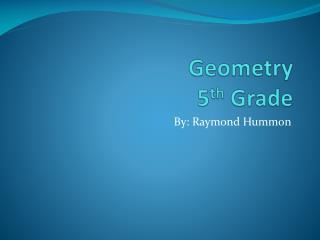DownloadDownload PresentationDownload Presentation- - - - - - - - - - - - - - - - - - - - - - - - - - - E N D - - - - - - - - - - - - - - - - - - - - - - - - - - -
##### Presentation Transcript

1. Geometry5th Grade By: Raymond Hummon

2. Polygon • What is a Polygon? • A Polygon is a closed plane figure bounded by three or more line segments.

3. N-Sided Polygons • 3 Sides- Triangle • 4 Sides- Quadrilateral • 5 Sides- Pentagon • 6 Sides- Hexagon • 7 Sides- Heptagon • 8 Sides- Octagon • 9 Sides- Nonagon • 10 Sides- Decagon

4. Triangle • Equilateral Triangle • Triangle with 3 equal sides • Isosceles Triangle • Triangle with 2 equal sides • Scalene Triangle • Triangle with 0 equal sides

5. Triangle cont. • Right Triangle • A right triangle has one 90° angle • Obtuse Triangle • An Obtuse triangle has one angle measuring more than 90° • Acute Triangle • An acute triangle has all three angles measuring less than 90° a piece

6. Quadrilateral • Quadrilaterals can be classified as well. • Different classifications include: • Square • Rectangle • Rhombus • Trapezoid • Kite • Parallelogram

7. QUIZ! • Here is the Podcast for your Quiz on Polygons.

8. Interactive Websites • http://www.kidskonnect.com/subject-index/17-math/291-geometry.html • http://www.kidsnewsroom.org/elmer/infocentral/geometry/index.html